×

Search anything:

# Time and Space complexity of Trie

#### Time Complexity trie Data Structures AlgorithmsIn this article, we will understand the Complexity analysis of various Trie operations. We have covered Time and Space Complexity of Trie for various cases like Best case, Average Case and Worst Case.

• Trie data structure
• Time and Space complexity of Trie operations
* Insertion
* Deletion
* Searching
• Conclusion

Pre-requisite: Trie data structure

To summarize, the time complexity is as follows:

Operation Average Case Worst Case Best Case
Insertion O(N) O(N) O(N)
Deletion O(N) O(N) O(N)
Searching O(N) O(N) O(1)

Space complexity for creation of a trie: O(alphabet_size * average key length * N)

The Space Complexity is as follows:

Operation Space Complexity
Insertion O(N)
Deletion O(1)
Searching O(1)

where:

• N is the average length of the keys in the trie.
• M is the extra nodes added

# Trie data structure

In computer science, Trie is a tree data structure which is used for dtoring collection of strings. In this data structure, strings that have common prefixes share an ancestor and hence it is also known as a prefix tree. It is maninly useful in storing dictionaries. We could even use hash trees for this purpose, but the main advantage of trie is that we do not need to perform an extra hash function to find out the position of the word and the words are not grouped together on the basis of their prefixes in a hash tree.

Each node in the trie has a boolen variable assigned to it that indicates whether that particular node is the end of the word or not. When its value is 'T', then that node is the end of the word, else it is not. There are many branches associated with each node of Trie which contains the possible character. Given below is a trie constructed for data {day,wa,way}.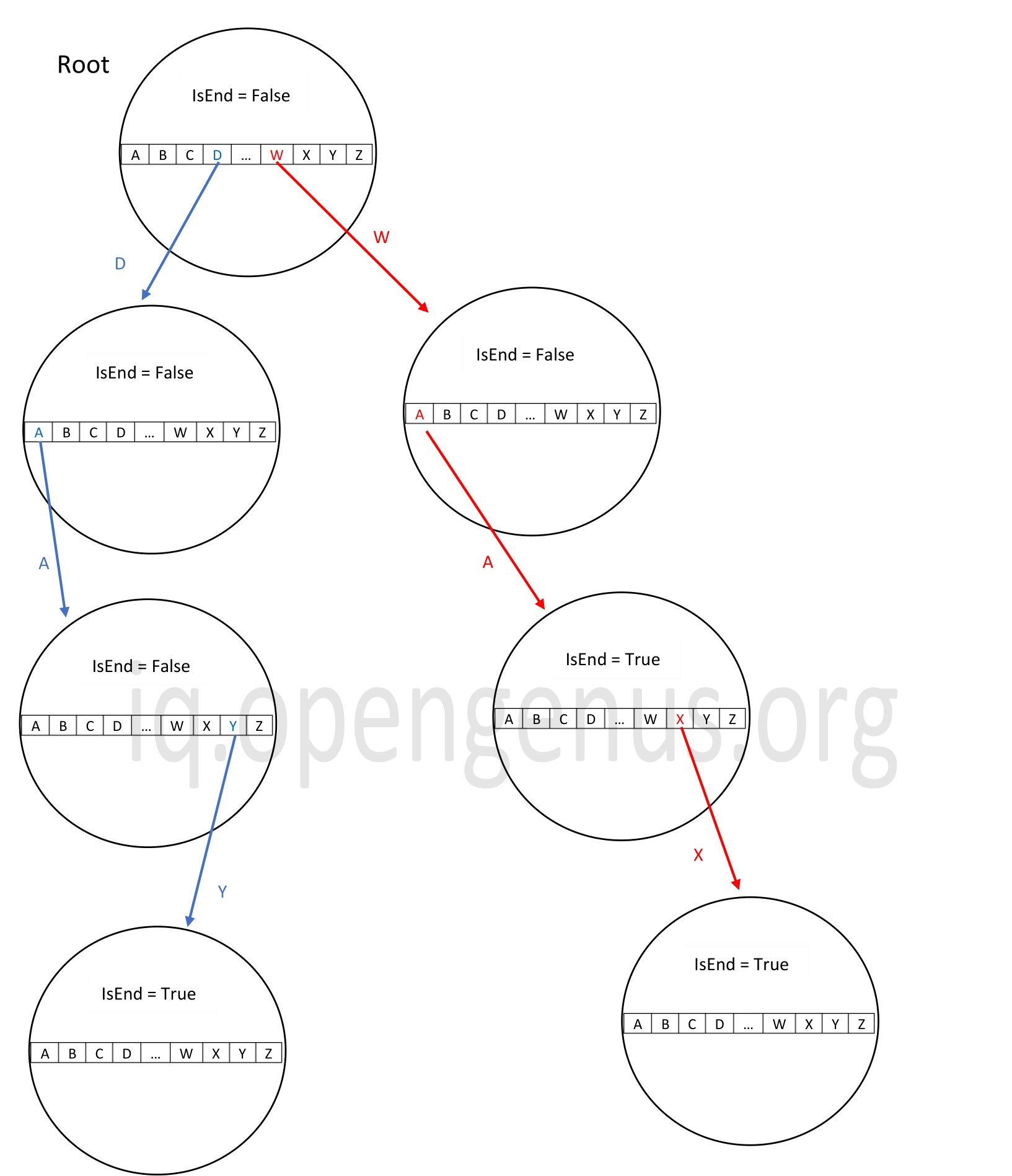We notice that in the above trie, the value of the IsEnd boolean variable is True after 'W','A' even though it then extends to 'X'. This is because, we have a word in the data 'wa' and it ends after 'A'. Now, since both 'wax'and 'wa' have a common prefix 'wa', they come under the same branch and 'X' is added in after 'A'.

# Time and Space complexity of Trie operations

The space complexity of creating a trie is O(alphabet_size * average key length * N) where N is th number of words in the trie.

Let us get into the details of complexity analysis of operations is Trie data structure.

## Insertion

While inserting into a trie, we first start from the root and see if any link for the first letter of the word inserted is already present. If it is already there, we then move down and search for the next character. If the link does not exist, we then create a new node and link it to the parent. If the key is not there in the root itself, then we create a new branch from the root that stores the alphabets in the word in the subsequent levels.

### Best case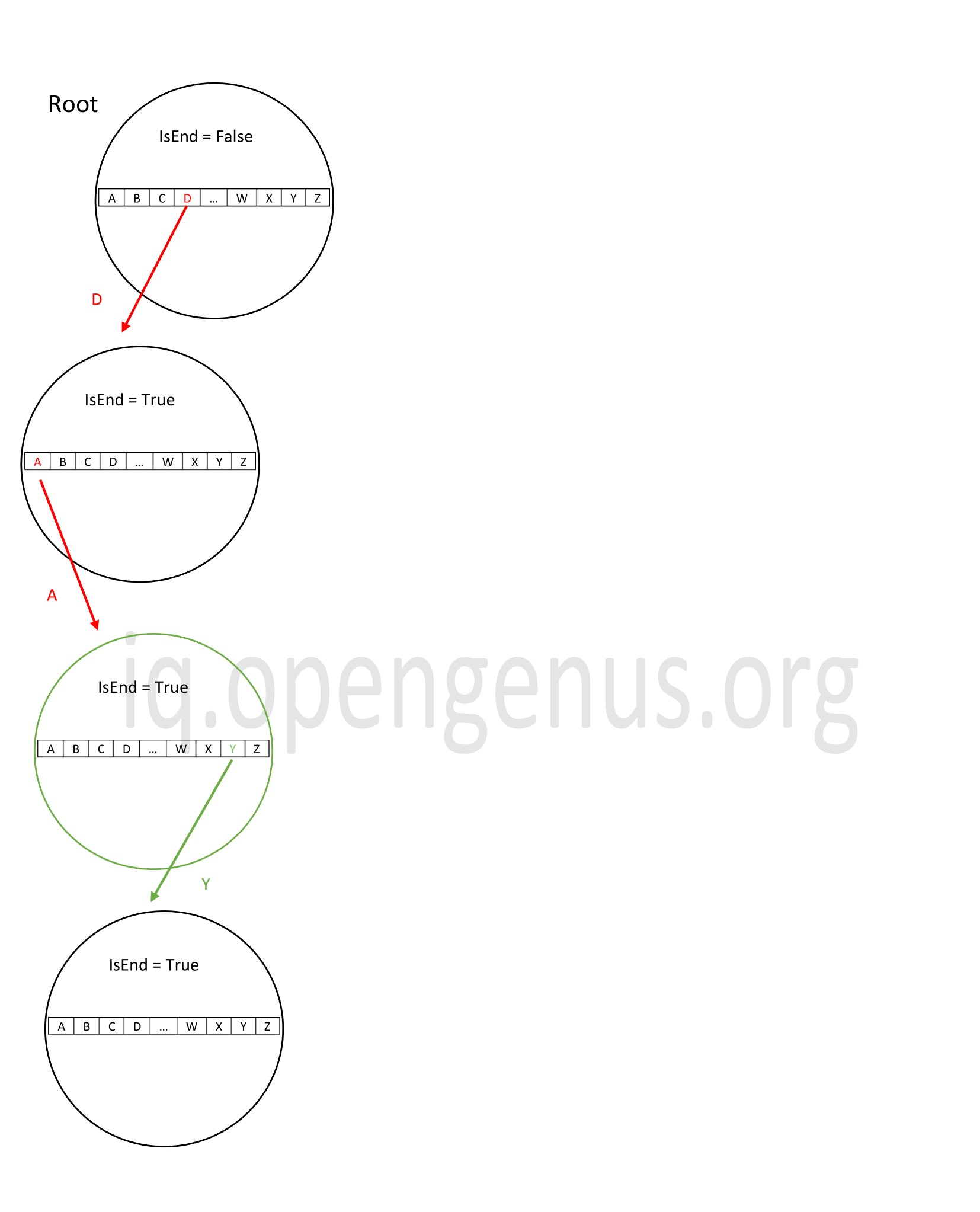In the above example, we are adding the word 'day' where strings 'd' and 'da' are already present in the trie. All words share the same prefix 'd'.

The time complexity in this case would be O(n) since we need to traverse down n nodes to insert the word 'day'. The space complexity will be O(m). Where m is the extra nodes added. In this case, it is just 'y'.

### Worst case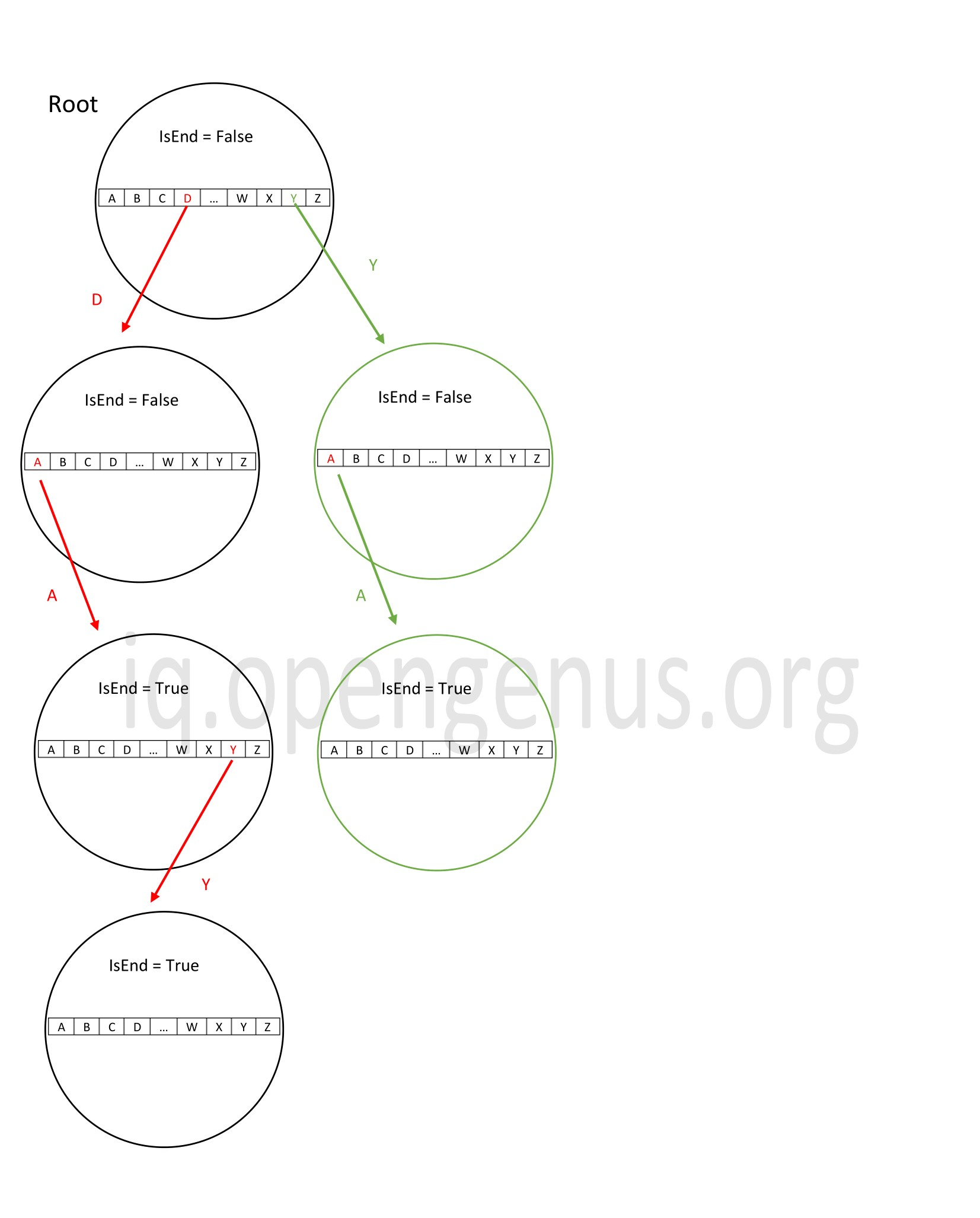In the above trie, we insert the string 'ya'. Since the link to 'y' is not available in the root itself, we create a new link and this word is inserted into the trie as a new branch.

Here, the time complexity will be O(n) where n is the length of the string to be inserted since we need to perform n iterations. The space complexity too will be O(n) where n is the length of the word since n new nodes are added which takes up space O(n).

The average case time complexity of insertion operation in a trie is too O(n) where n is the average length of the keys in the trie.

## Deletion

While performing deletion, we delete keys in a trie from bottom to top using recursion. Deletion should be performed in such a way that the other strings in the trie are not affected.

### Best case

The best case deletion is when the word to be deleted is a prefix of another word. So that no node is deleted and just the boolen variable is changed to remove that particular word from the trie.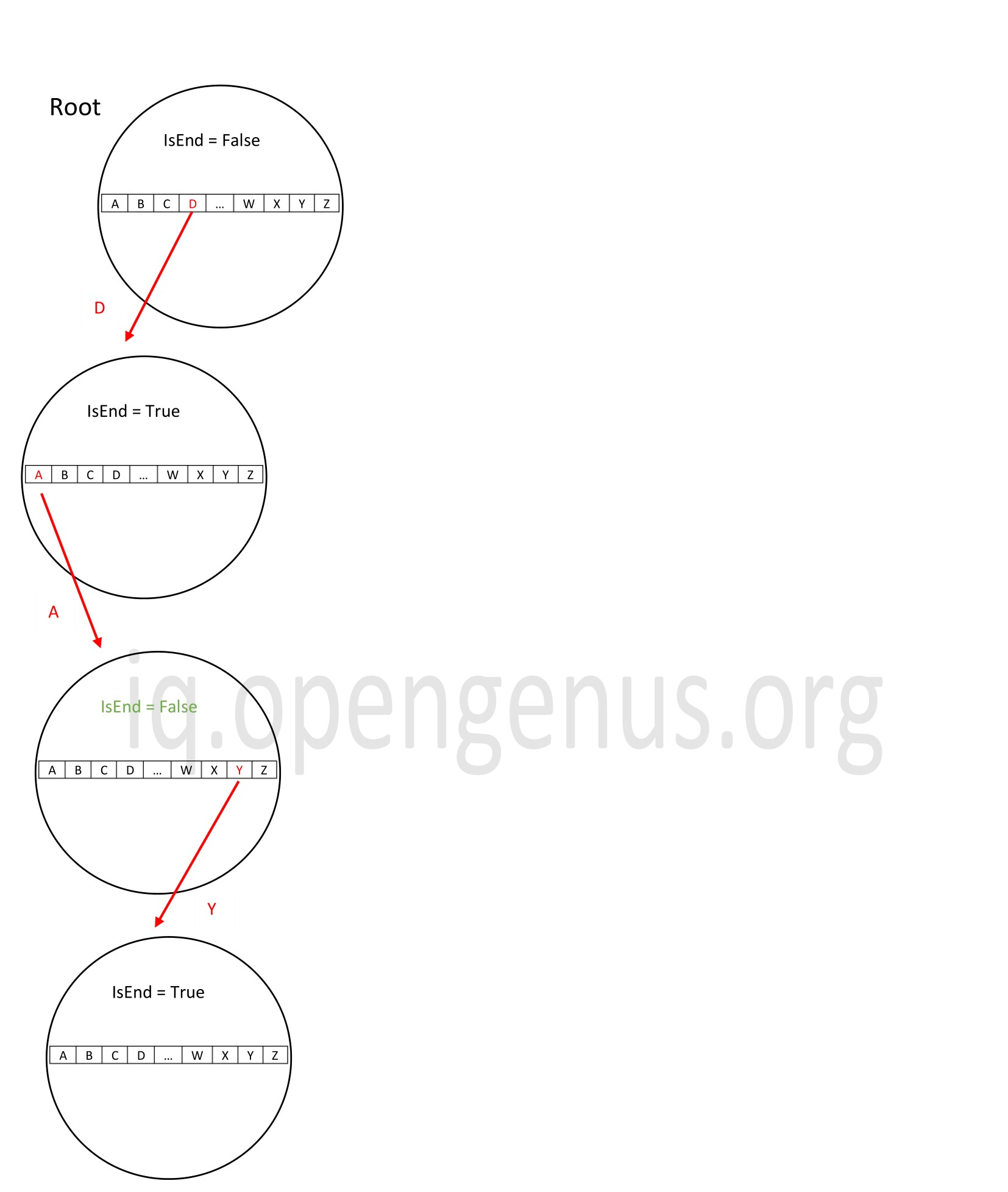In the above trie, we delete the string 'da'. To achieve this, we traverse the length of the word and reach 'a'. We change the boolean value after 'da' to false. Thus the sting 'da' is deleted without disturbing the other words in the trie.

The time complexity in this case is O(n) where n is the length of the string to be deleted since we need to traverse down its length to reach the leaf node.

### Worst case

The worst case deletion is when the whole string to be deleted is a unique string which does not share its nodes with any other string i.e. a separate branch. In this case, each node of that word is to be deleted.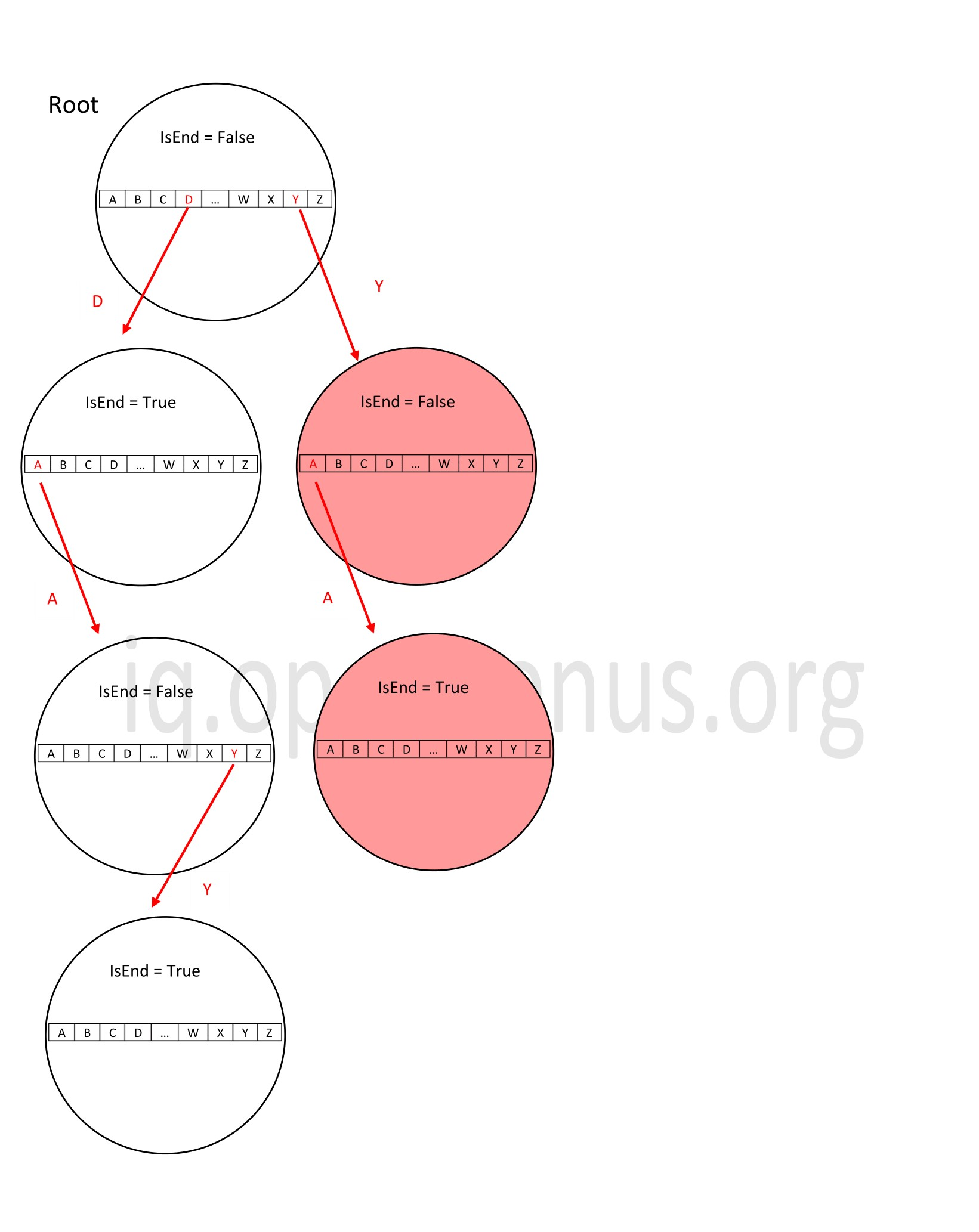In the above example, the word 'ya' is to be deleted. We notice that the string 'ya' forms a separate branch in the trie and when we want to delete it, we must delete every node of it.

The time complexity in this case is O(n) where n is the length of the string to be deleted since we need to traverse down its length to reach the leaf node.

The average case time complexity of deletion operation in a trie is too O(n) where n is the average length of the keys in the trie.

## Searching

Search operation in a trie is the same as insert. The algorithm looks for the key and is it is present, it recursively moves down to its child and is terminated only when the word ends or there is no such word present in the trie.

### Best case

The best case search is when the key to be searched is present in the root itself and we need not traverse down further.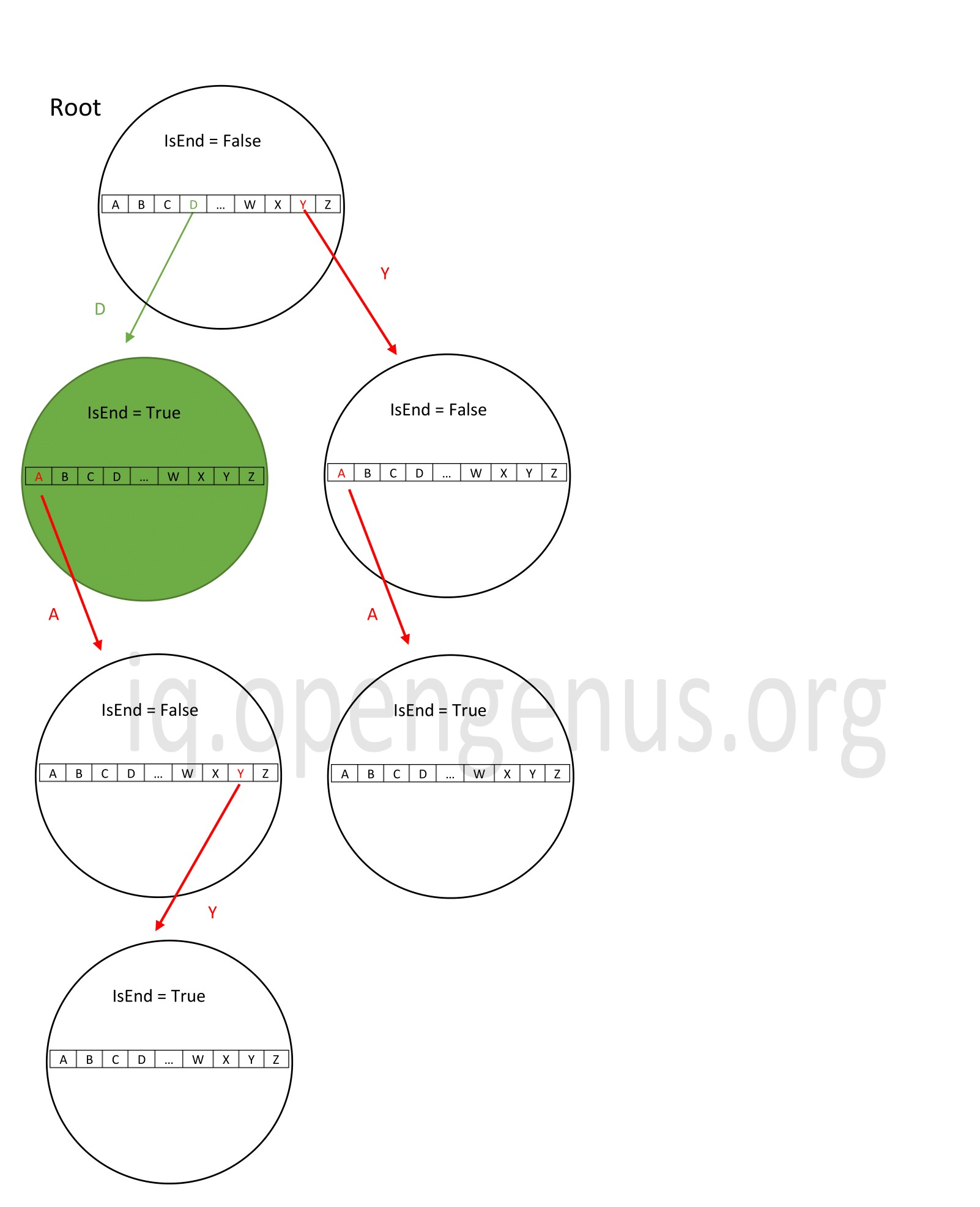In the above example, the trie consists of data { d, day, ya} and we search for 'd'. We find it in the first iteration itself.

The time complexity in this case will be O(1) since we find the word after just a single pass.

### Worst case

The worst case search is when we need to traverse the length if the word to find it in the trie.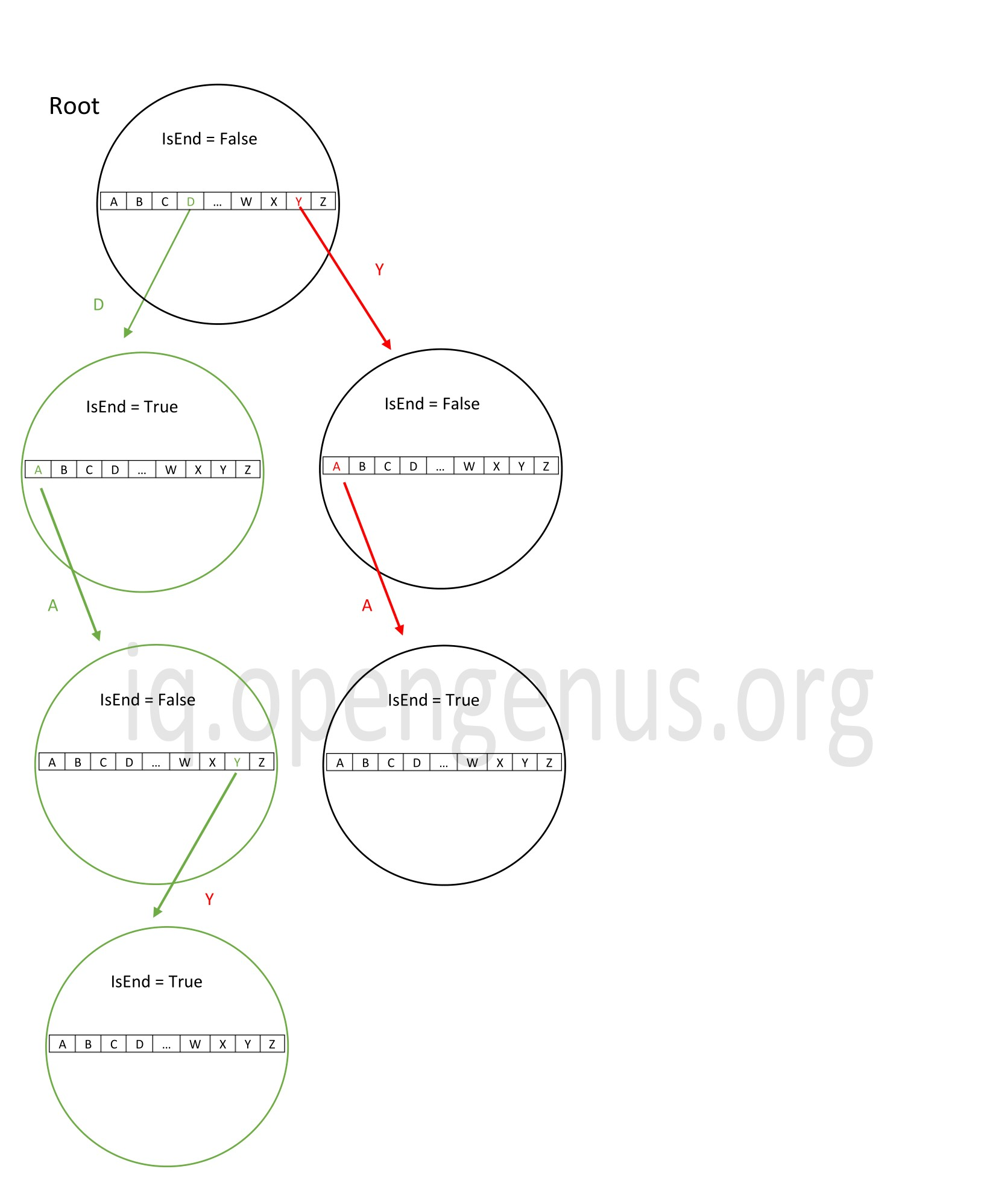Now in the same trie, if we want to search for the word 'day', we need to travel down the length of it ( the path taken is denoted in green).

In this case, the time complexity will be O(n) where n is the length of the word to be searched.

The average case time complexity of searching operation in a trie is too O(n) where n is the average length of the keys in the trie.

# Conclusion

To summarize, the time complexity is as follows:

Operation Average Case Worst Case Best Case
Insertion O(N) O(N) O(N)
Deletion O(N) O(N) O(N)
Searching O(N) O(N) O(1)

Space complexity for creation of a trie: O(alphabet_size * average key length * N)

The Space Complexity is as follows:

Operation Space Complexity
Insertion O(N)
Deletion O(1)
Searching O(1)

where:

• N is the average length of the keys in the trie.
• M is the extra nodes added

With this article at OpenGenus, you must have the complete idea of Time and Space Complexity of Trie data structure.#### Sanjana Babu

Sanjana Babu is a Student at SRM Valliammai Engineering College and is an Intern at OpenGenus. She is a Philomath.

Time and Space complexity of Trie